# Combine the SUBSTITUTE and LEN functions### Combine the SUBSTITUTE and LEN functions

Tips: you can turn on the volume by clicking

Here is a form recording the personal information of staff. How can we calculate the staff number for each department?

We can combine the LENS and SUBSTITUTE functions to realize it.

Before starting, let me introduce the LEN function. It can return the character number of the text.

Select cell A4. Go to the formula editing box for the Insert Function button, and choose the LENS function.

Since we want to know the character number in cell A2, we need to enter A2 as the TEXT parameter.

Click OK. Then, the result returns as 11. Note that each punctuation such as a comma(,), a sentence stop(.), and a space( ), are counted as one character.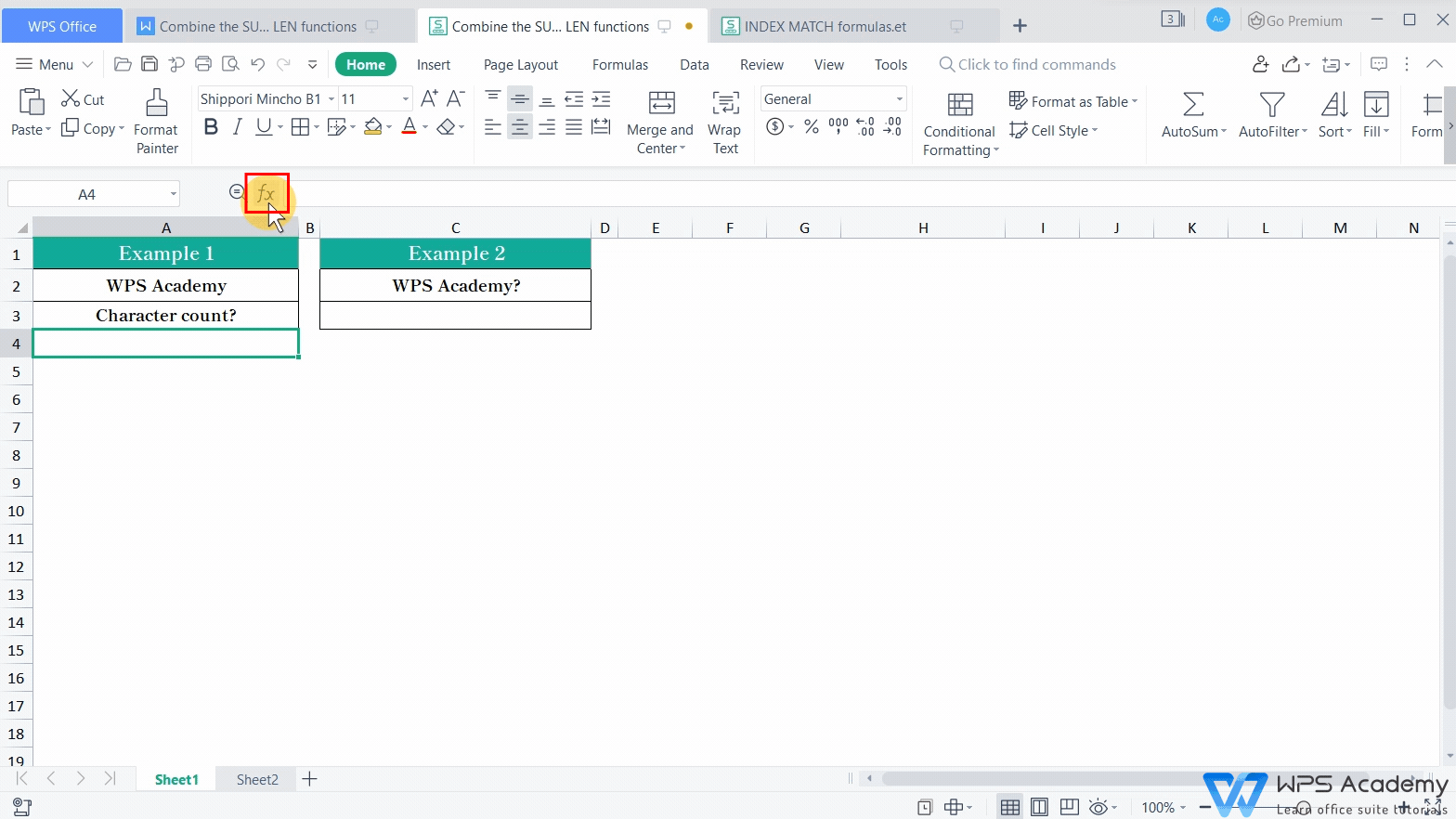Next, it's the introduction to the SUBSTITUTE function. The SUBSTITUTE function is used to replace the old text with the new text.

For example, we can use it to remove all question marks(?) in cell C2. Here are the steps.

1. Select cell C3, click the Insert Function button and insert the SUBSTITUTE function.

2. Choose the cell for operation as the TEXT parameter. Here we choose C2.

3. Enter the content to be replaced as the Old_text parameter.

Finally, enter a pair of quotation marks () as the New_text parameter, which means removing the Old Text.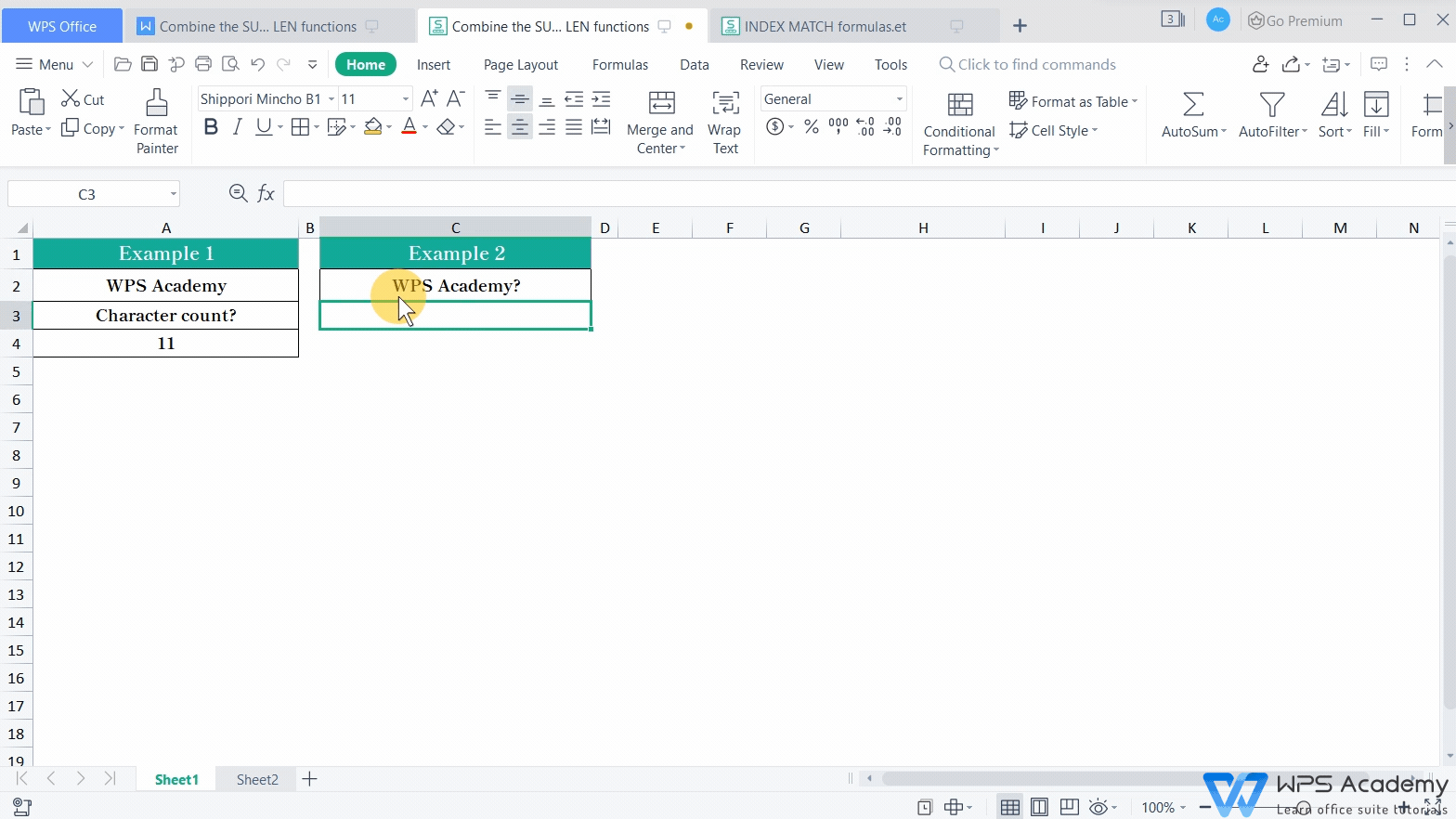In the previous part, we've learned about the LEN and the SUBSTITUTE functions. In the following part, we'll count the staff numbers by combining these two functions.

From cell B2, we can find that a comma is inserted after each staff's name. So, to be simple, if we can get the comma number, we'll know the staff number. Note that since there's no comma after the last staff's name, the actual amount of staff equals the amount of comma plus one.

Here are the steps to get the result.

First, we use the LEN function to find out the number of characters for all employee names in cell B2. In order to avoid taking into account the number of characters for commas, we need to nest the SUBSTITUTE function after the LEN function. And, we can use the SUBSTITUTE function to delete all commas in the text.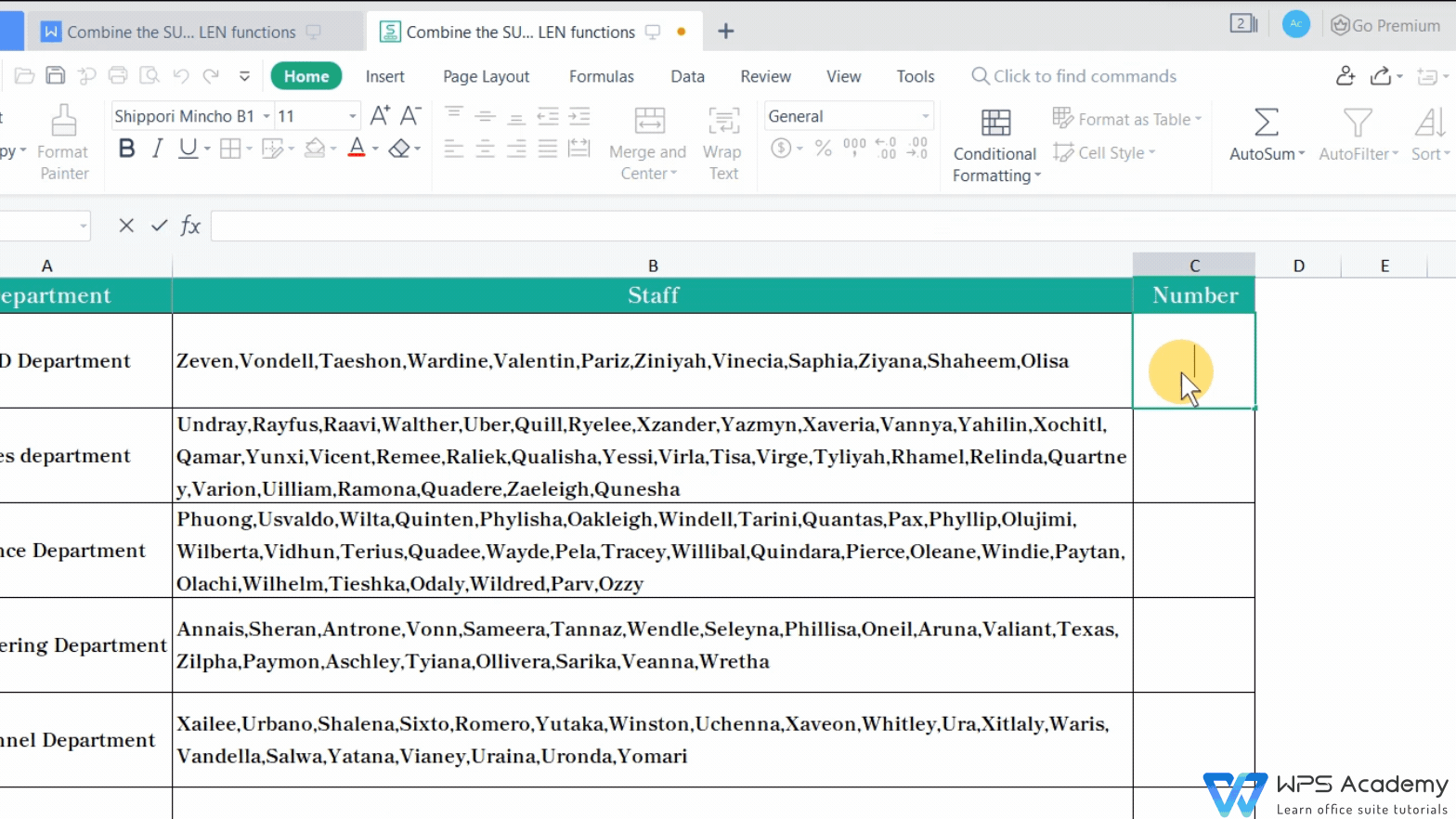Second, we can use the total number of characters in cell B2 to subtract the value just calculated by the nested function.

Third, at the end of the formula, enter “+1”.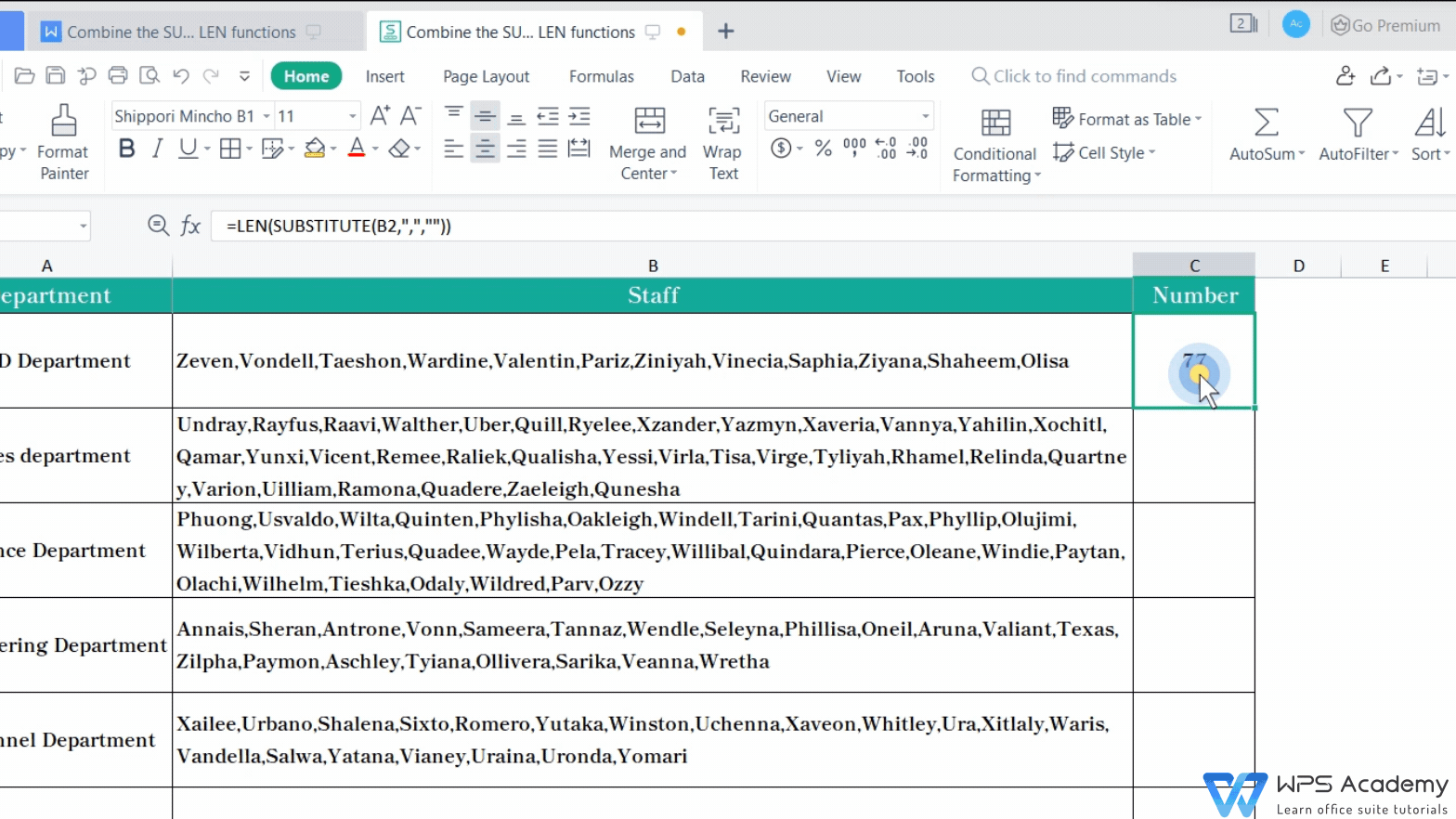The result will return as 12.

Finally, use the AutoFill function to get the staff numbers for all departments.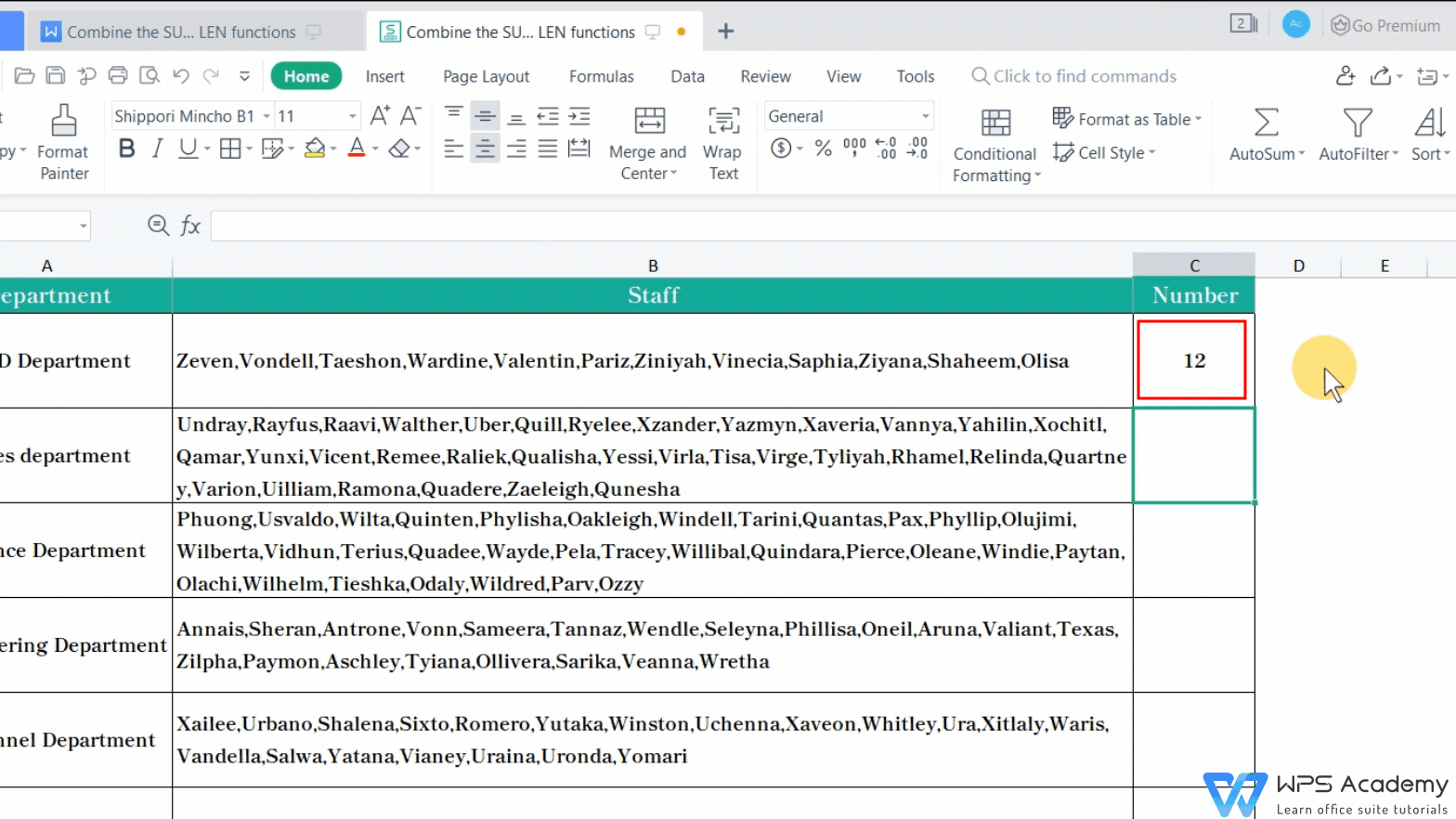Here's the end of the tutorial. Did you get it?

To be office excel advanced, you could learn how to use WPS Office Spreadsheet online in WPS Academy.